Constructing perpendicular to the line segment

Practical Geometry
Serial order wise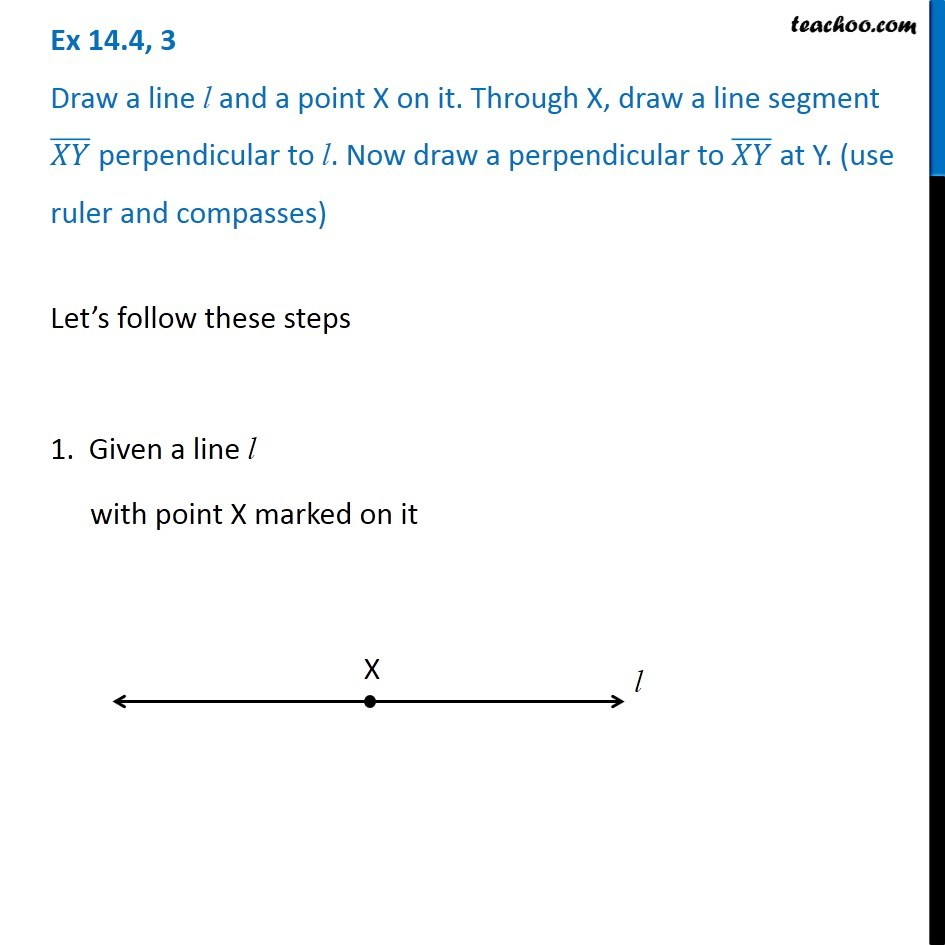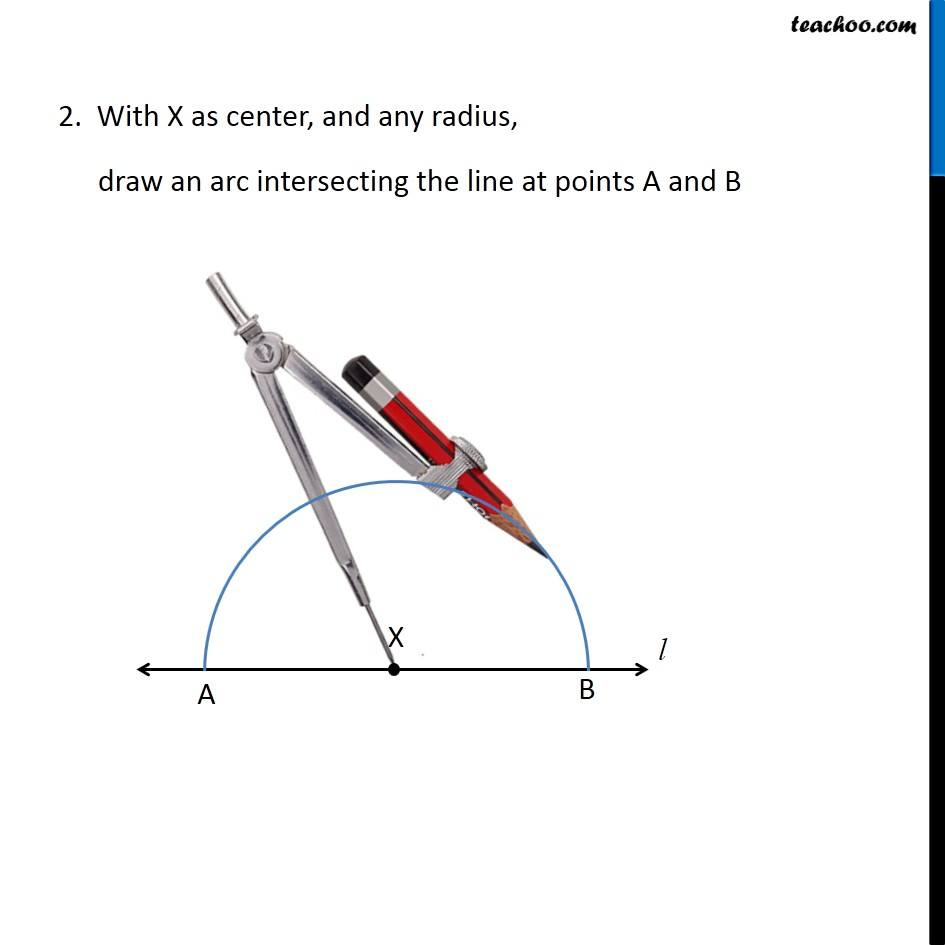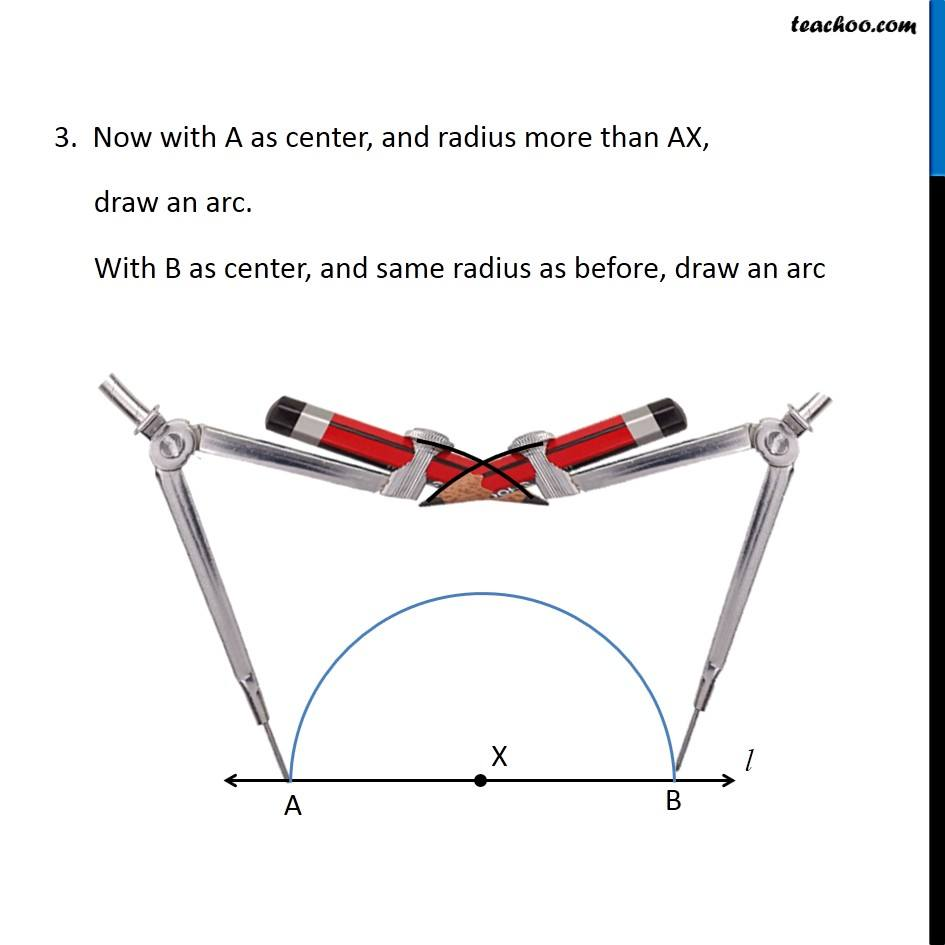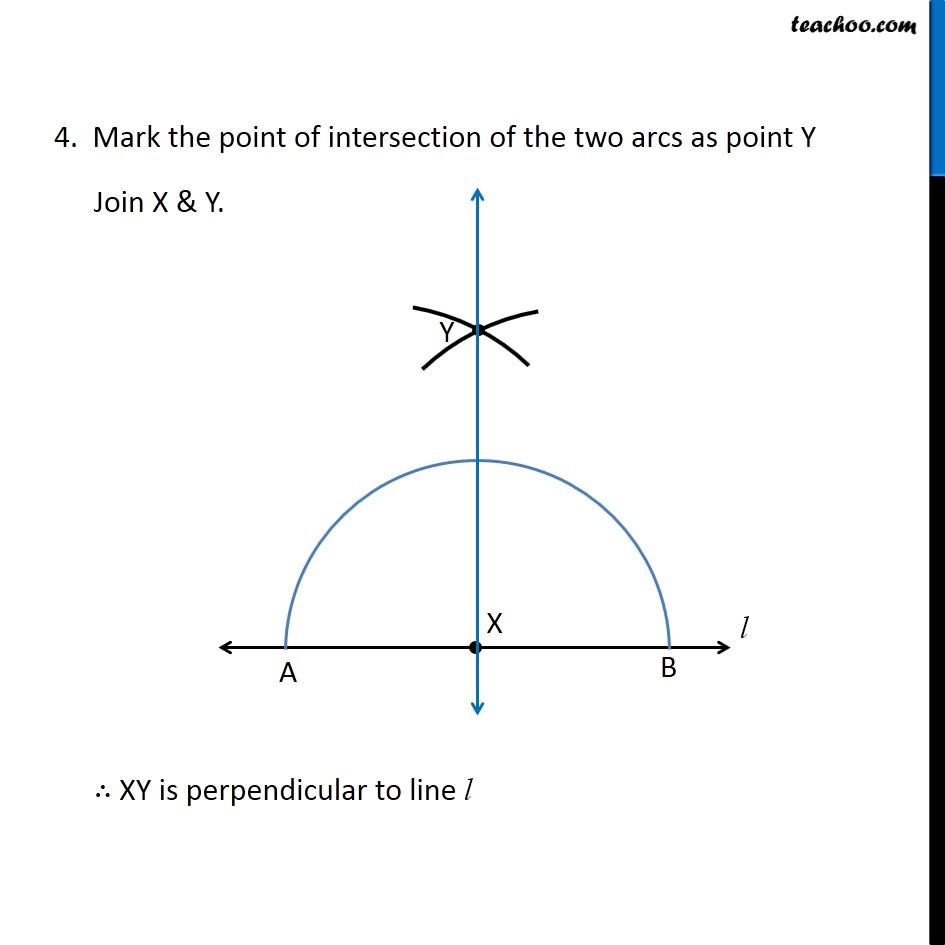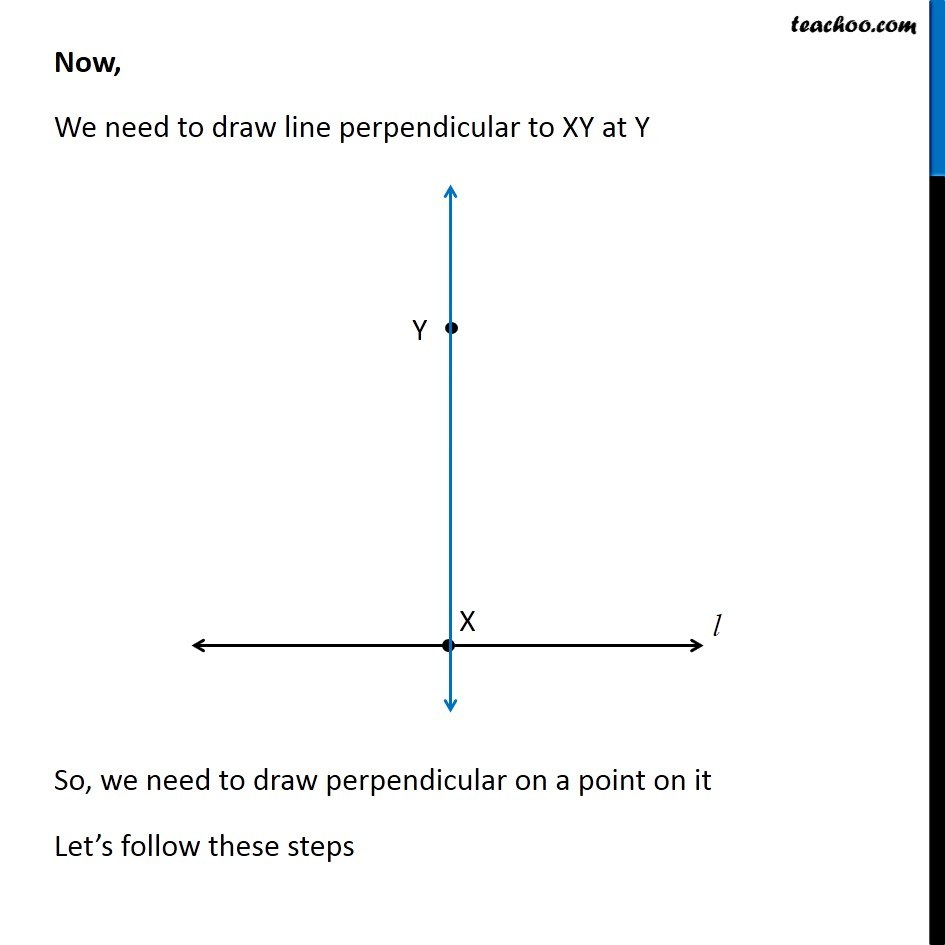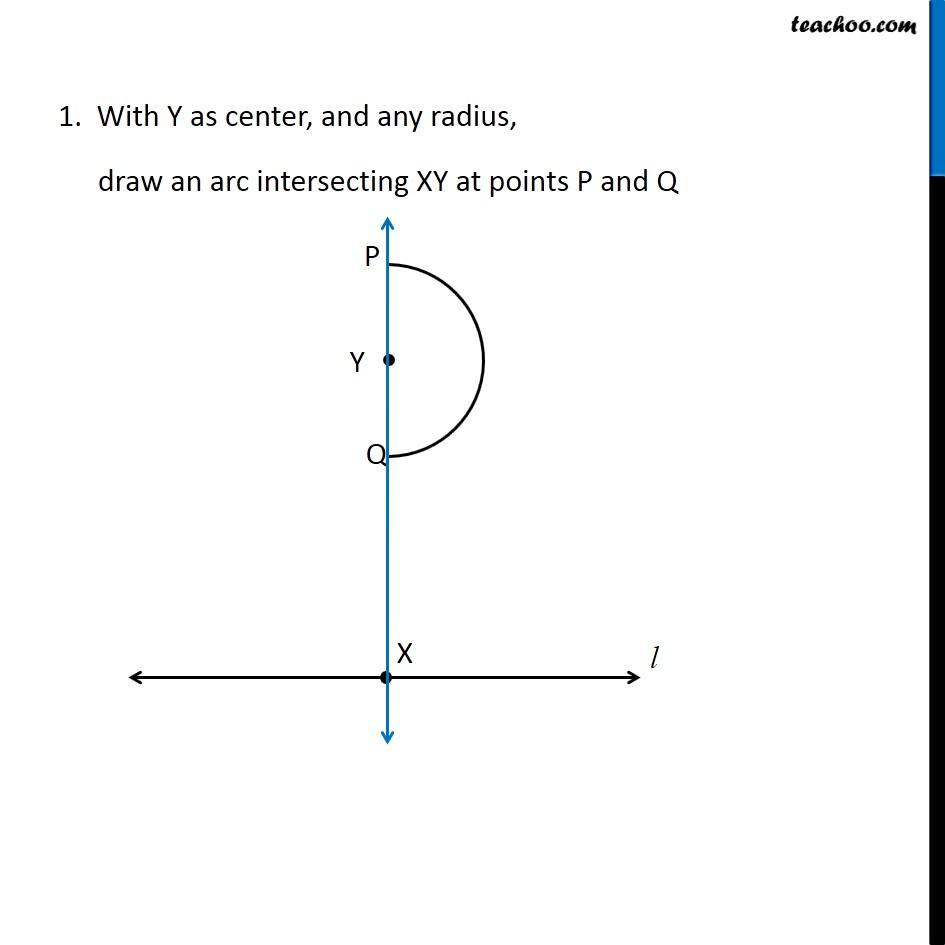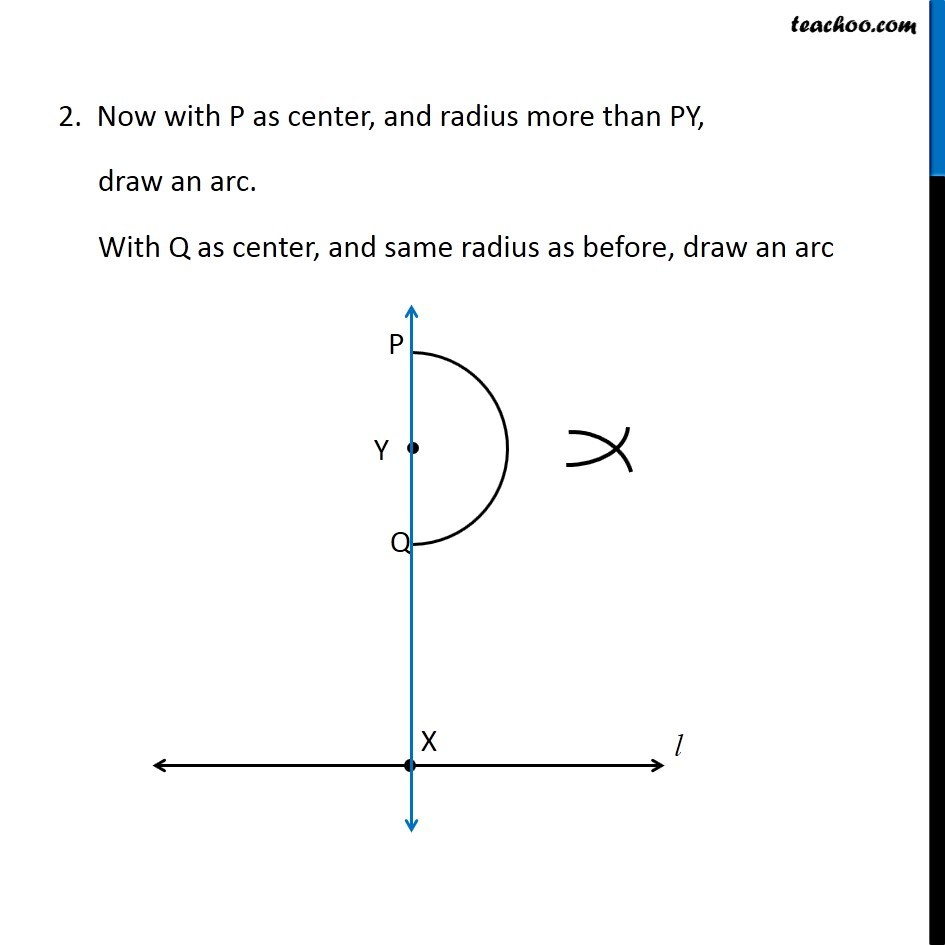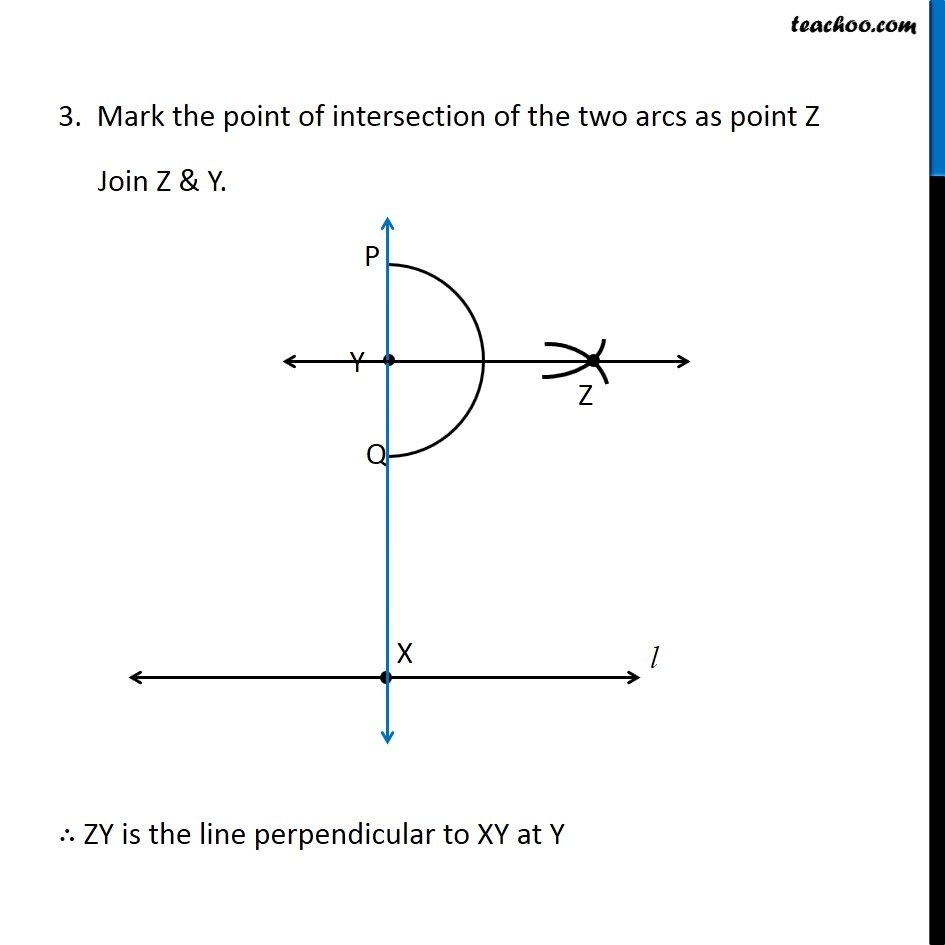Learn in your speed, with individual attention - Teachoo Maths 1-on-1 Class

### Transcript

Question 3 Draw a line l and a point X on it. Through X, draw a line segment (𝑋𝑌) ̅ perpendicular to l. Now draw a perpendicular to (𝑋𝑌) ̅ at Y. (use ruler and compasses) Let’s follow these steps 1. Given a line l with point X marked on it Let’s follow these steps 1. Given a line l with point X marked on it 3. Now with A as center, and radius more than AX, draw an arc. With B as center, and same radius as before, draw an arc 4. Mark the point of intersection of the two arcs as point Y Join X & Y. ∴ XY is perpendicular to line l Now, We need to draw line perpendicular to XY at Y So, we need to draw perpendicular on a point on it Let’s follow these steps 1. With Y as center, and any radius, draw an arc intersecting XY at points P and Q Now with P as center, and radius more than PY, draw an arc. With Q as center, and same radius as before, draw an arc 3. Mark the point of intersection of the two arcs as point Z Join Z & Y. ∴ ZY is the line perpendicular to XY at Y